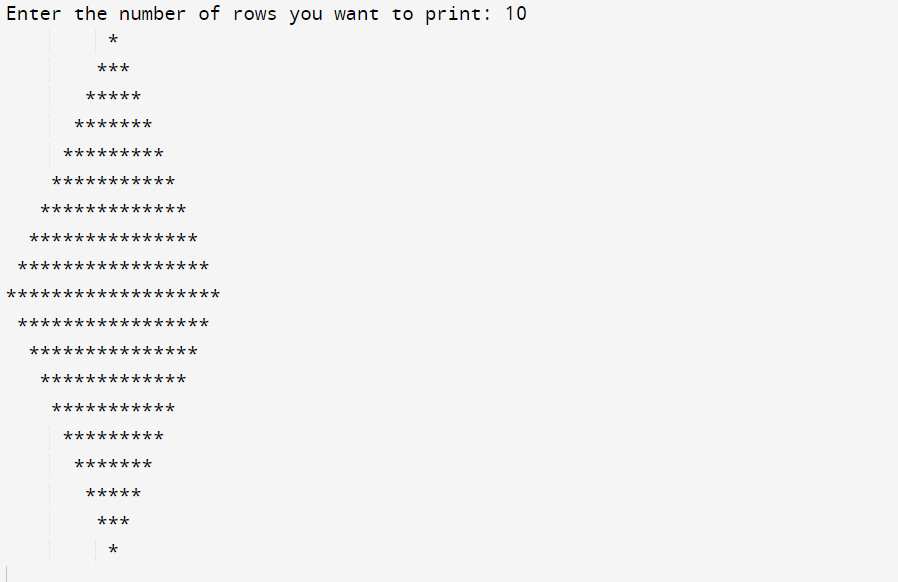top of page
Search

# Write a Program to print Diamond Star Pattern in Java

The diamond star pattern consists of asterisks (*) arranged in a diamond shape. The pattern has a central row with a single asterisk, and the number of asterisks on each subsequent row increases until reaching the midpoint of the pattern. After reaching the midpoint, the number of asterisks decreases on each row until a single asterisk is reached again.

In this article, we will provide you with the proper code which will print the Diamond Star pattern in Java with a proper explanation of the code.

### Code to print Diamond Star Pattern in Java:

```import java.util.Scanner;
public class DiamondPattern
{
public static void main(String args[])
{
int row, i, j, space = 1;
System.out.print("Enter the number of rows you want to print: ");
Scanner sc = new Scanner(System.in);
row = sc.nextInt();
space = row - 1;

for (j = 1; j<= row; j++)
{
for (i = 1; i<= space; i++)
{
System.out.print(" ");
}
space--;

for (i = 1; i <= 2 * j - 1; i++)
{
System.out.print("*");
}
System.out.println("");
}
space = 1;

for (j = 1; j<= row - 1; j++)
{
for (i = 1; i<= space; i++)
{
System.out.print(" ");
}
space++;

for (i = 1; i<= 2 * (row - j) - 1; i++)
{
System.out.print("*");
}
System.out.println("");
}
}
}  ```

Let's break down the code and understand how the above code will print the diamond star pattern:

1. The user is prompted to enter the number of rows they want to print for the diamond pattern.

2. The program initializes a variable space to row - 1, which represents the number of spaces to be printed in each row. This variable is used to control the indentation of the pattern.

3. The first loop for (j = 1; j <= row; j++) is responsible for printing the upper half of the diamond. It iterates from j = 1 to j = row.

4. Inside this loop, the program uses a nested loop for (i = 1; i <= space; i++) to print the spaces before the asterisks. The number of spaces decreases as i increases, resulting in indentation.

5. After printing the spaces, the program enters another nested loop for (i = 1; i <= 2 * j - 1; i++). This loop prints the asterisks (*) in each row. The number of asterisks is given by 2 * j - 1. As j increases, the number of asterisks also increases.

6. After printing the spaces and asterisks for each row, a newline is printed using System.out.println(""), which moves the cursor to the next line.

7. The program then resets the space variable to 1 for the lower half of the diamond.

8. Another loop for (j = 1; j <= row - 1; j++) is executed to print the lower half of the diamond. It iterates from j = 1 to j = row - 1.

9. Inside this loop, the program uses a nested loop for (i = 1; i <= space; i++) to print the spaces before the asterisks. Similar to the upper half, the number of spaces increases as i increases.

10. After printing the spaces, the program enters another nested loop for (i = 1; i <= 2 * (row - j) - 1; i++) to print the asterisks. The number of asterisks is given by 2 * (row - j) - 1. As j increases, the number of asterisks decreases.

11. Finally, the pattern is printed in the console, consisting of the upper half and lower half of the diamond shape.

### Output:### Conclusion

Creating patterns using programming can be an engaging and rewarding exercise. In this article, we explored how to write a Java program to print a diamond star pattern. By employing loops and control structures, we achieved the desired symmetrical shape. Understanding and implementing such patterns can enhance your understanding of loop structures and enable you to tackle more complex programming challenges. So go ahead, give it a try, and have fun exploring the world of pattern printing in Java!